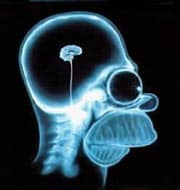# Science without sense…double nonsense

## Having a large n, who needs a small p?

This post is also available in: Spanish

The cult of p is one of the most widespread religions in Medicine. His believers always look for the p-values when reading a scientific paper and feel great devotion when they see that p is very small, full of zeros.

But, in recent times, it has emerged a serious competitor to this cult: the worshipers of n which, as we all know, represents the sample size. Because it happens that with currently available information tools, it’s relatively easy to perform studies with large sample sizes. Well, you might think, we can combine the two faiths in one and worship those studies that, with huge sample sizes, get very tiny values of p. The problem is that it leads us away from what should be our true religion, which must only be the assessing of the size of the observed effect and its clinical relevance.

When we observe a difference in effect between the two arms of a trial we must ask whether this difference is real or simply due to chance. What we do is set up a null hypothesis that the difference is due to chance and calculate a statistical value that gives us the probability that the difference is, in fact, random. This value is the statistical significance, our p. The p-value only indicates the probability that the difference is due to chance. By convention, we usually set the limit to 0.05, so when p is less than this value we consider reasonably likely that the difference is not due to chance, so we consider that the effect actually exists.

The p-value that we can get depends on various factors such as the dispersion of the variable we are measuring, the effect size, and the sample size. Small samples are more imprecise so that p-values, keeping all other factors unchanged, are smaller the larger the sample size.

Imagine that we compared the values of blood pressure reduction with two different drugs in a clinical trial, and we get a mean difference between the groups of 5 mmHg. If the test includes 20 patients, p-value may not be significant (being greater than 0.05), but it is likely that this same difference becomes significant if the trial engaged 10,000 patients. Indeed, in many cases reaching statistical significance may be only a matter of increasing the sample size. This is why very large samples can get significance with very small effect sizes. In our example, a confidence interval for mean difference of 1 to 6 mmHg is statistically significant (not including zero, the null value for mean differences), although the effect is probably insignificant from a clinical point of view. The difference is real, although the clinical significance may be nonexistent.

In summary, any effect, however slight, can be statistically significant if the sample is large enough. Let’s see an example with the Pearson’s correlation coefficient, R.

The minimum correlation coefficient to reach statistical significance (p <0.05) for a given sample size will be equal to two divided by the square root of the sample size (I will not show it mathematically, but you can calculate it from the formulas for calculating the 95% confidence interval of R).

This means that if n = 10, any value of R> 0.63 is statistically significant. Well, you will say, 0.63 is an acceptable value to establish a correlation between the two variables value, it may imply some interesting clinical meaning. If we calculate R2, it has a value of 0.4, which means that 40% of the variability of the dependent variable is explained by changes in the independent. But think for a moment what would happen in n = 100,000. Any value of R > 0.006 will be significant, even with a p-value with many zeroes. And what do you think about a R value of 0.006?. Indeed, it probably won’t be much relevant no matter its statistical significance as the amount of variability of one variable explained by the variability of the other will be negligible.

The problem that arises in practice is that it is much more difficult to define the limits of clinical relevance than that of statistic significance. As a general rule, the effect is statistically significant when the confidence interval does not include the null value. On the other hand, it will be clinically relevant when some of the values within the interval are considered relevant by the investigator.

And here we end for today. Just a little comment before we finish. I simplified a bit the reasoning of the relationship between n and p, exaggerating the examples to prove that large samples can be so discriminative that the value of p loses some of its interest. However, there are times when this is not so. The value of p depends greatly on the size of the smallest group analyzed, so when the studied effect is rare or one of the groups is very small, our p-values regain its prominence and its zeros become again useful. But that’s another story…

This site uses Akismet to reduce spam. Learn how your comment data is processed.